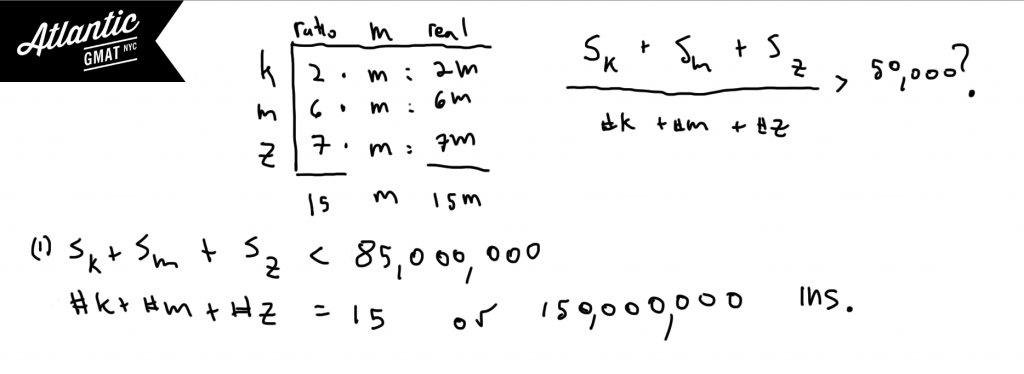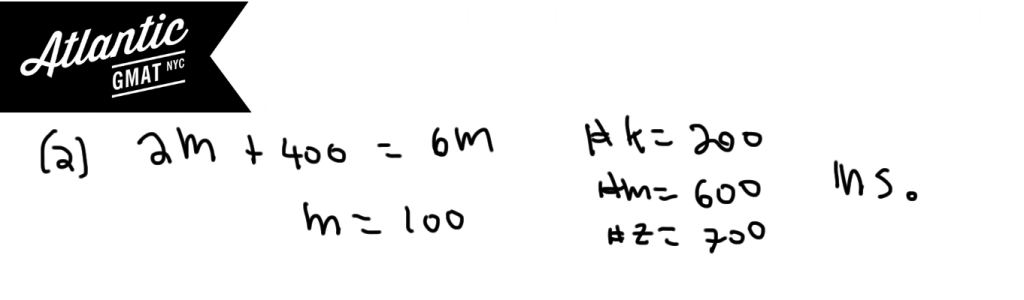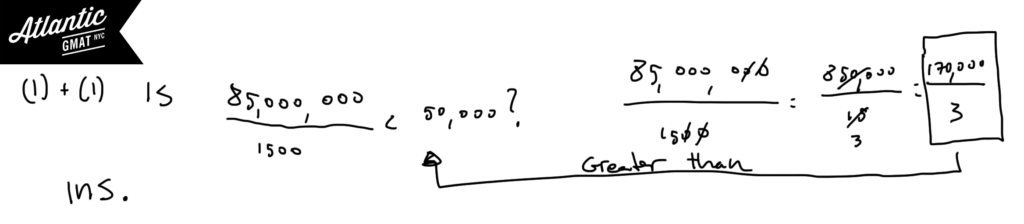GMAT Question of the Day - Data Sufficiency - Ratio

The ratio of the number of employees at company's K, M, and Z is 2 to 6 to 7. Is the average salary at these companies less than \$50,000?

1. The total earnings at all three companies is less than \$85,000,000

2. Company K has 400 fewer employees than company M does

GMAT Question of the Day Solution

This is a tricky ratio question but certainly not out of bounds of what you will see on a real GMAT. The concepts tested here are important and find their way in many a GMAT question easy and hard. The main topic is weighted average. This question mixes in some ratio as well. It is important to understand the components of average (total, # of things, and the average itself). Define these components. It can be useful to organize them in a T (similar to a rate/work T). This is also a threshold question with the threshold being at \$50,000. Below that the answer is yes. Above that and the answer is no. On both sides and the statement is insufficient. So the main thing that you need to know is where are you relative to the threshold?

Statement (1) tells you the top limit of the total salary. The range of the salary is from 0 to \$85,000,000. Even though this is an enormous range it still could be sufficient if the top of the range provided an average below \$50,000.

But since we don't know how many employees there are splitting this salary we can create averages above and below \$50,000. You can test this with extremes. The fewest employees is 15. That would give you the largest average (clearly above 50,000 per employee). You could also choose a gigantic number of employees (I chose 150 million). This gives you an average salary far below 50,000. Because you can be on either side of the threshold this statement is insufficient.Statement (2) gives you the number of employees. By itself this is insufficient because you don't have any information on the salary.Statement (1) + (2) Putting them together still doesn't answer the question because the upper limit is still above 50,000 and the lower limit can go down to zero. The greatest possible average salary is 170,000/3 which is greater than 50,000. The least possible average is zero (clearly below 50,000). Because you can answer yes and no this is insufficient.Sorry, comments are closed for this post.

CONTACT

Atlantic GMAT

405 East 51st St.

NY, NY 10022

(347) 669-3545

info@AtlanticGMAT.com